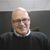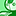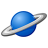Announcements
Sept. 27, 10AM ET Do more with Qlik, Insight Advisor – our intelligent AI-assistant in Qlik Sense: Register
cancel
Showing results for
Did you mean:Partner - Contributor II

## Set expression problem

Hi All,

Set expression is not working properly ,it is giving zero values.

vperiod-SubField(PeriodWeek,'-',2)

Exp: sum({<Period={"\$(vperiod)"}>}Sales)

Please find the attached app an data.

Muni

15 RepliesPartner - Champion III

To get something that works I believe you have to do this:

Sum( {<UniqueRowID={"=Period=SubField(PeriodWeek,'-',2)"}>} Sales)

The UniqueRowID is as the name implies a field that uniquely identifies each and every row in the table that contains the Sales field.

If you don't have a candidate for that in the table already you will have to create one. It is easy to do in your load script by creating a calculated field in the load statement of the table that contains the Sales field:

SALES:

RowNo() AS Sales.uid,

Sales,

.....

If you do the expression would be changed to:

Sum( {<Sales.uid={"=Period=SubField(PeriodWeek,'-',2)"}>} Sales)Partner - Contributor II
Author

can we get same result with out new fieldCreator III

try this:

vperiod1    =mid(PeriodWeek,6,3)

and

expression as:

if(Match(mid(PeriodWeek,6,3),'03','06','09','12'

),

sum({<Period={"\$(vperiod1)"}>}Sales)/5,

sum({<Period={"\$(vperiod1)"}>}Sales)/4

)Partner - Champion III

As I mentioned - if you already have a field in the table that contains the Sales field that is unique across the rows you can use that and  you don't need a new field.

Look at the Excel data all the rows have the  Period equal to the subfield of the PeriodWeek so I don't understand what you are trying to achieve with this set expression?Partner - Creator III

The set analysis expressions are evaluated once per chart so

{<Period={"\$(vperiod)"}>}

does not work because you want a different value of the variable vperiod for each value of the dimension (PeriodWeek). Why dont you use an IF statement?

If( Match(Only({1<Week>}Right(SubField(PeriodWeek,'-',2),2)),'03','06','09','12'),

sum(if(Period=\$(vperiod), Sales))/5,
sum(if(Period=\$(vperiod), Sales))
/4
)

check the attached App.qwv if you prefer!

Bests,

Jaime.Partner - Contributor II
Author

i have some other filters in the set expression not only period.Partner - Creator III

You can keep that filters except the period and include the IF:

If( Match(Only({1<Week>}Right(SubField(PeriodWeek,'-',2),2)),'03','06','09','12'),

sum( {< OTHER FILTERS>} if(Period=\$(vperiod), Sales))/5,
sum( {< OTHER FILTERS>} if(Period=\$(vperiod), Sales))/4
)Partner - Contributor II
Author

it is not filter the data by above expression.can you please try some other exp.Specialist